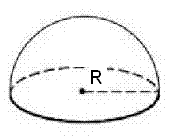# Hemisphere Calculator

Calculate the volume, curved surface area(CSA) and total surface area(TSA) of hemisphere. The hemisphere is exactly half of a sphere. When a plane cuts across a sphere at its center then it forms two hemispheres.Volume of Hemisphere: [ (2/3)πr³ ]
 Enter the Radius = Volume of Hemisphere =Curved Surface Area(CSA) of Hemisphere: [ 2πr² ]
 Enter the Radius = Curved Surface Area(CSA) of Hemisphere =Total Surface Area(TSA) of Hemisphere: [ 3πr² ]
 Enter the Radius = Total Surface Area(TSA) of Hemisphere =

The Surface Area of Hemisphere is the area occupied by the surface of the solid object and it is classified into two types as follows:
1. Curved Surface Area (CSA)
2. Total Surface Area (TSA)The Curved Surface Area of Hemisphere is the half part of a sphere, therefore the curved surface area is also half of that sphere.

Curved surface area (CSA) = 1/2 ( 4 π r2) = 2 π r2
The Base Area is the base of the hemisphere in circular shape. As we know by the formula of area of circle as follows:
Area of base = π r2
To calculate the Total Surface Area of the hemisphere, we need to consider the base of the hemisphere which is circular. Therefore, it is equal to:
Total Surface Area (TSA) = Curved Surface Area + Area of the Base Circle
= 2 π r2 + π r2
= 3 π r2